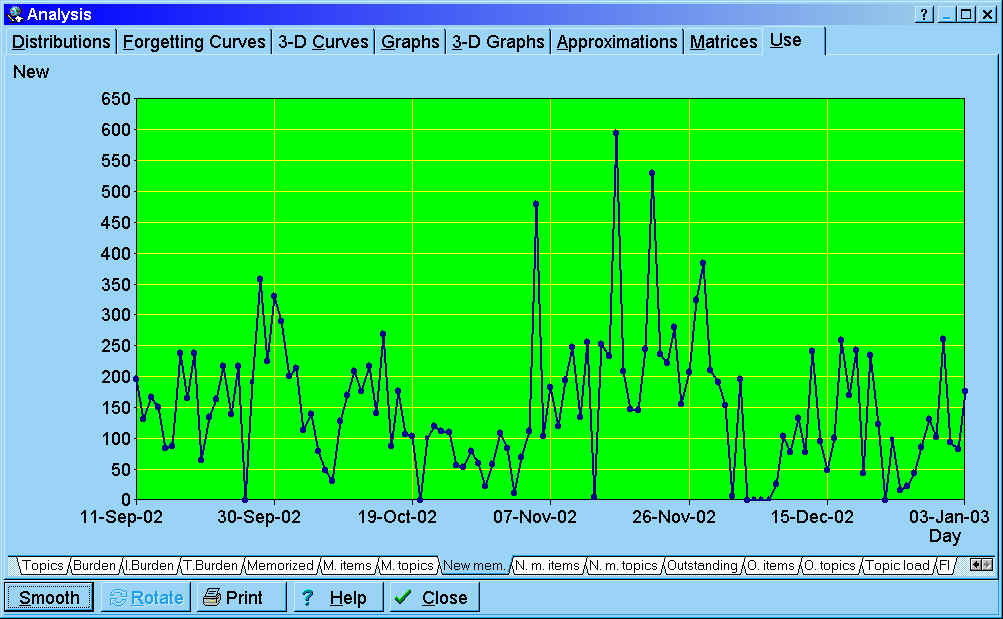## Analysis of the learning process

The tabbed dialog available with Tools : Statistics : Analysis in SuperMemo provides graphs and tables that illustrate the current state of the learning process in the currently opened collection.

The following tabs are available in the Analysis dialog box:

Distributions

Interval distribution - distribution of inter-repetition intervals in a given collection. By changing the setting of the combo box at the bottom of the dialog, you can see interval distributions for topics, items and for all elements in the collection

A-Factor distribution - distribution of A-Factors in a given collection. The distribution itself is not used in Algorithm SM-11, and merely results from it. Note that topics and items use different A-Factor categories. Choose Items only or Topics only at the bottom to see distributions for items or topics. If you choose, All elements, you will see a less meaningful conglomerate distribution indexed by A-Factor category number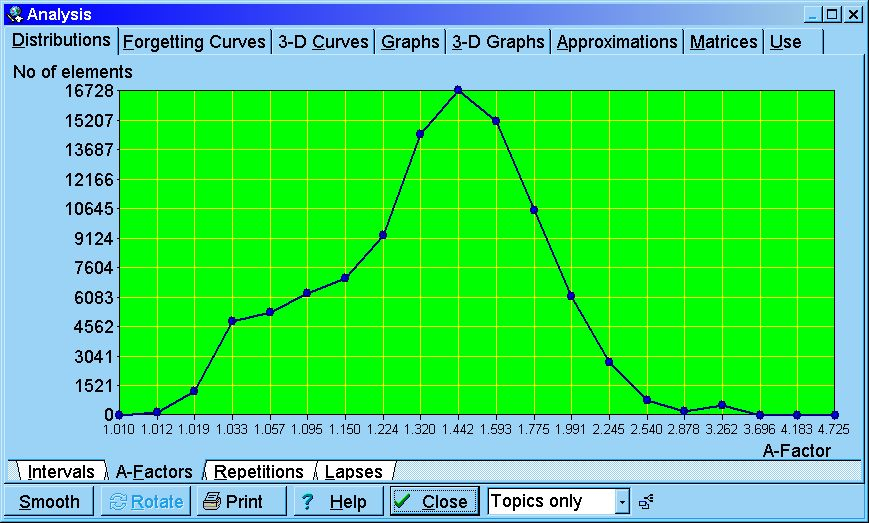Repetitions distribution - distribution of the number of repetitions of items or topics in a given collection (only memorized elements are considered in the distribution, i.e. there is no zero-repetitions category)

Lapses distribution - distribution of the number of times particular elements in the collection have been forgotten (only memorized elements are considered). As topics are never 'forgotten', there are no lapses listed in Lapses distribution if you select Topics only in the element type combo box

Forgetting Curves - 400 forgetting curves are independently plotted in order to compute the RF matrix in Algorithm SM-11. These correspond to twenty repetition number categories multiplied by twenty A-Factor categories (note that for data representation convenience, the columns of the RF matrix for the first repetition are indexed by the number of memory lapses rather than A-Factor). By choosing a proper combination of tabs at the bottom of the graph, you can select a forgetting curve of interest. Horizontal axis represents time expressed as: (1) U-Factor, i.e. the ratio of subsequent inter-repetition intervals, or (2) days (only for the first repetition). Vertical axis represents knowledge retention in percent.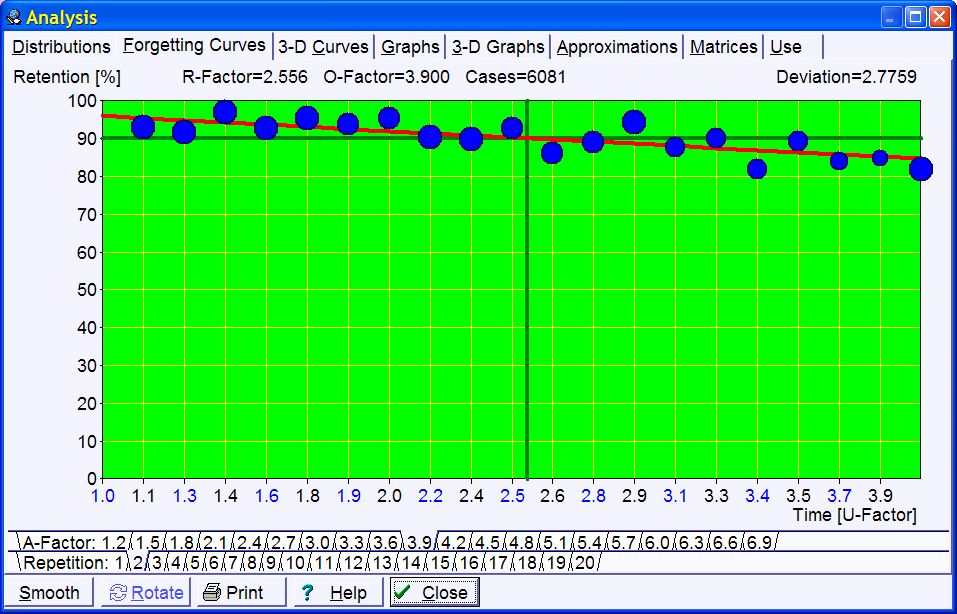Blue circles in the picture represent data collected during repetitions. The greater the circle, the greater the number of repetitions recorded. The red curve corresponds with the best-fit forgetting curve obtained by exponential regression (note that for ill-structured material the forgetting curve is crooked, i.e. not exactly exponential). The horizontal green line corresponds with the requested forgetting index, while the vertical green line shows the moment in time in which the approximated forgetting curve intersects with the requested forgetting index line. This moment in time determines the value of the relevant R-Factor, and indirectly, the value of the optimum interval (for the first repetition, R-Factor corresponds with the first optimum interval). The values of O-Factor and R-Factor are displayed at the top of the graph. They are followed by the number of repetition cases used to plot the graph. At the beginning of the learning process, there is no repetition history and no repetition data to compute R-Factors. It will take some time before your first forgetting curves are plotted. For that reason, the initial value of the RF matrix is taken from the model of a less-than-average student. The model of average student is not used because the convergence from poorer student parameters upwards is faster than the convergence in the opposite direction.

3-D Curves

These graphs show the forgetting curves in 3 dimensions. Each graph corresponds with a different A-Factor (from 1.2 to 6.9). The X axis represents time (as in Forgetting curves). The Y axis represents repetition category. The vertical Z axis represents retention in percent. For better visibility, you can rotate 3-D forgetting curves with Rotate, or smooth them with Smooth. Note that smoothing proceeds along the Y axis. This makes it possible to align incompletely plotted forgetting curves from different repetition categories. However, once you click Rotate, Y axis smoothing will produce a different result. For the best illustration of the forgetting process, smooth the graph before you rotate it.

Graphs

Forgetting Index vs. Priority graph - this graph shows you how forgetting depends on the priority of the learning material. If you always go through your outstanding material in time and if you stick to the 20 rules of knowledge formulation, your measured forgetting index should be the same as the requested forgetting index. This graph will then show a flat straight line passing horizontally at the level of the forgetting index. However, once you start overloading the learning process and using Postpone or Mercy, your measured forgetting index will increase. If you always sort your repetitions by priority (e.g. by leaving Learn : Sorting : Auto-sort repetitions checked), you should still ensure that your measured forgetting index equals the requested forgetting index for high priority elements (i.e. on the left side of the graph). Your measured forgetting index for low priority material will be higher, and the greater the overload the greater the difference. If you change your repetition sorting criteria by increasing randomization of repetitions, this graph will be flatter and the measured forgetting index for high priority material will be higher

First interval graph - the length of the first interval after the first repetition depends on the number of times a given item has been forgotten. Note that the first repetition here means the first repetition after forgetting (i.e. not the first repetition ever). In other words, a twice repeated item will have the repetition number equal to one after it has been forgotten (i.e. the repetition number will not equal three). The first interval graph shows exponential regression curve that approximates the length of the first interval for different numbers of memory lapses (including the zero-lapses category that corresponds with newly memorized items). In the graph below, blue circles correspond to data collected in the learning process (the greater the circle, the more repetitions have been recorded). In the presented case, newly memorized items are optimally repeated after seven days; however, items that have been forgotten 10 times (which is rare in SuperMemo) will require the interval of two days (due to logarithmic scaling, the size of the circle is not linearly proportional to the data sample and the number of repetition cases for lapses=0 is by far larger than for lapses=10 as can be seen in Distributions : Lapses distribution). The graph below includes data from over 130,000 repetitionsD-Factor vs. A-Factor graph - DF-AF graph shows decay constants of power approximation of R-Factors along columns of the RF matrix. You need to understand SuperMemo Algorithm to understand this graph. The horizontal axis represents A-Factor, while the vertical axis represents D-Factor (i.e. Decay Factor). D-Factor is a decay constant of power approximation of curves that can be inspected with the Approximations tab of the Analysis dialog box

First Grade vs. A-Factor graph - G-AF graph correlates the first grade obtained by an item with the ultimate estimation of its A-Factor value. At each repetition, the current element's old A-Factor estimation is removed from the graph and the new estimation is added. This graph is used by the SuperMemo Algorithm to quickly estimate the first value of A-Factor at the moment when all we know about an element is the first grade it has scored in its first repetition

Grade vs. Forgetting Index graph - FI-G graph correlates the expected forgetting index with the grade scored at repetitions. You need to understand SuperMemo Algorithm to understand this graph. You can imagine that the forgetting curve graph might use the average grade instead of the retention on its vertical axis. If you correlated this grade with the forgetting index, you would arrive at the FI-G graph. This graph is used to compute the estimated forgetting index that in turn is used to normalize grades (for delayed or advanced repetitions) and estimate the new value of item's A-Factor. The grade is computed using the formula: Grade=Exp(A*FI+B), where A and B are parameters of exponential regression run over raw data collected during repetitions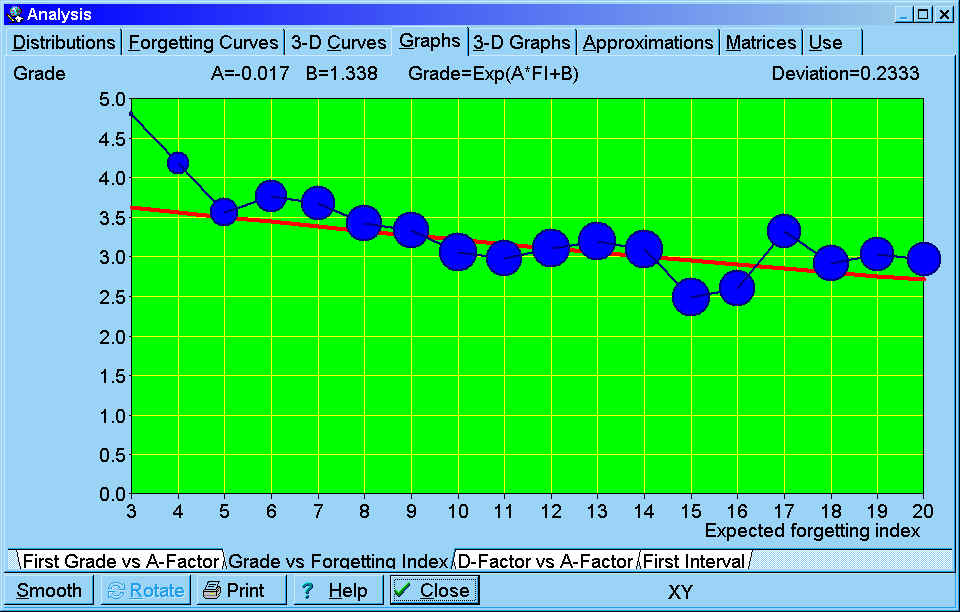3-D graphs that visually represent OF, RF and Cases matrices (see: Matrices)

Approximations

Twenty power approximation curves that show the decline of R-Factors along columns of the RF matrix. You need to understand SuperMemo Algorithm to understand these graphs. For each A-Factor, with increasing values of the repetition number, the value of R-Factor decreases (at least theoretically it should decrease). Power regression is used to illustrate the degree of this decline that is best reflected by the decay constant called here D-Factor. By choosing the A-Factor tab at the bottom of the graph, you can view a corresponding R-Factor approximation curve. The horizontal axis represents the repetition number category, while the vertical axis represents R-Factor. The value of D-Factor is shown at the top of the graph. The blue polyline shows R-Factors as derived from repetition data. The red curve shows the fixed-point power approximation of R-Factor (fixed-point approach is used as for the repetition number equal two, R-Factor equals A-Factor). The green curve shows the fixed-point power approximation of R-Factor derived from the OF matrix. This is equivalent to substituting the D-Factor obtained by fixed-point power approximation of R-Factors with D-Factor obtained from DF-AF linear regression

Matrices - you need to understand SuperMemo Algorithm to understand these tables.

O-Factor matrix - matrix of optimal factors indexed by the repetition number and A-Factor (only for the first repetition, A-Factor is replaced with memory lapses)

R-Factor matrix - matrix of retention factors

Cases matrix - matrix of repetition cases used to compute the corresponding entries of the RF matrix (double click an entry to view the relevant forgetting curve). This matrix can be edited manually (e.g. if you want to change the weight of some measurements during repetitions)

Optimal intervals - matrix of optimum intervals derived from the OF matrix

D-Factor vector - vector of D-Factor values for different A-Factor values (also repetition cases used in computing particular D-Factors)

Use

Graphs of changes in the statistics of the learning process over time. These graph record daily changes to statistics such as the number of memorized items, measured forgetting index or the number of outstanding items for a given day. The first non-zero value of the day is recorded. If that value increases afterwards, that increase is ignored (except a few cases such as the statistic of newly memorized items, etc.). If you have a break in learning, the last recorded non-zero value will propagate through the period in which you did not use SuperMemo (except a few cases such as the statistics of repetitions made, etc.).

To zoom in on a portion of the graph (as in pictures below), sweep the portions of the graphs that are to be removed with the mouse (e.g. point the day of focus, move the mouse left and release the mouse button). You can also use Ctrl+Left and Ctrl+Right buttons to zoom in.

Here are the statistics recorded daily by SuperMemo and displayed on the Use tab:

1. Work done
• Use time - your daily total time in which you actively used a given collection (as recorded in Tools : Timeline)
• Recall time - your daily total time which you spent on trying to recall answer to questions
• Repetitions - your daily recorded number of item and topic reviews
• Item repetitions - your daily record of item repetitions
• Topic repetitions  - your daily record of topic reviews
2. Outstanding - number of outstanding elements scheduled for a given day
• Outstanding - number of all outstanding elements scheduled for a given day
• Outstanding items - number of outstanding items scheduled for a given day
• Outstanding topics - number of outstanding topics scheduled for a given day
3. Burden
• Burden - current Burden parameter record. Burden estimates the number of elements scheduled for repetition daily on the assumption of no delays in the learning process. Burden is the sum of interval reciprocals. Burden = Item burden + Topic burden
• Item burden - current Burden for items
• Topic burden - current Burden for topics
4. Overload - the number of executed repetitions divided by the number of outstanding repetitions (i.e. the percent of the actually executed repetitions)
• Elements - the percent of all outstanding repetitions executed
• Items - the percent of outstanding item repetitions executed
• Topics  - the percent of outstanding topic reviews done
5. Element count
• Elements - total number of elements in the collection as recorded on a given day (i.e. topics + items + tasks)
• Items  - number of items in the collection as recorded on a given day
• Topics and tasks  - number of topics and tasks in the collection as recorded on a given day
6. Memorized
• Memorized - number of elements taking part in the learning process (i.e. not dismissed and not pending)
• Memorized items - number of items taking part in the learning process
• Memorized tasks - number of topics taking part in the learning process
7. Newly memorized
• Newly memorized - new elements memorized on a given day (see an exemplary graph below)
• New items - new items memorized on a given day
• New topics - new topics memorized on a given day
8. Efficiency
• Forgetting index - measured forgetting index
• Retention - retention as measured on each day
• Topic load - proportion of topics scheduled for review on a given day among all elements scheduled

Exemplary graph showing the total number of topics stored in the collection. The data displayed on the bottom depends on the position of the mouse. In this example, it indicates that on Jul 20, 2003, there were 104,604 topics in the incremental reading process.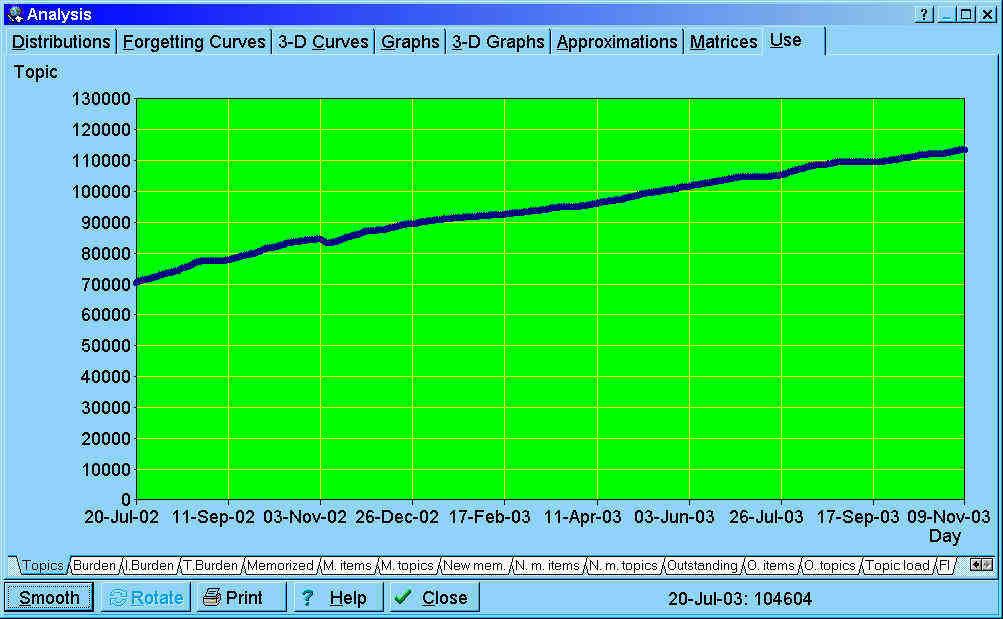Exemplary graph showing the number of elements memorized on individual days. By sweeping away the left and right part of the graph, it is possible to zoom in on a small section of the learning process. The period shown on the graph spans from Sep 11, 2002 to Jan 3, 2003.